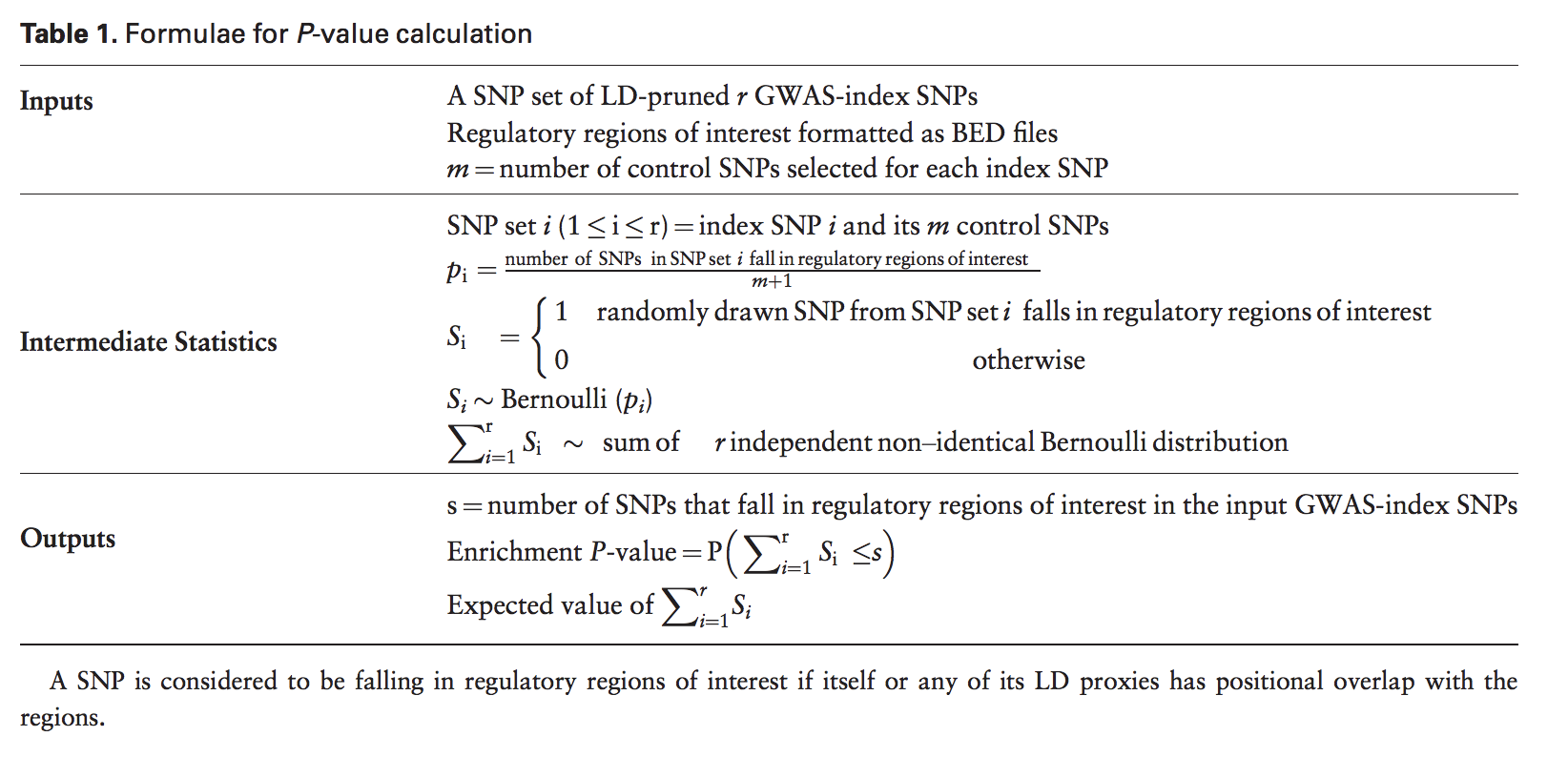GREGOR

1. Create a list of potentially function variants.
• Index SNPs + LD friends (\(r^2>0.7\)) using the 1000 Genomes data.
1. Overlap this list of SNPs with various cell type-/tissue-specific annotations.

2. Calculate the total number of trait-associated loci at which either the index SNP or one of its LD proxies overlaps with an annotation.

3. Assess significance of this overlap by estimating the probability of the observed overlap of GWAS SNPs relative to expectation using a set of matched control variants.
1. For each GWAS index SNP, identify a set of ~500 control SNPs randomly selected from across the genome that match the index SNP for (i) number of variants in LD, (ii) MAF and (iii) distance to nearest gene.
2. Assume that the number of index SNPs within its matched control set of SNPs that overlaps a given feature follows a binomial distribution with \(n=\) the number of GWAS index SNPs present in the control set and \(p=\) the proportion of SNPs within the control set or their LD proxies that physically overlaps a feature
3. Compute the sum of independent binomial random variables
4. For each regulatory feature, calculate the fold-enrichment over expectation and an enrichment P value that represents the probability that the overlap of control SNPs represented as a cumulative probability distribution is greater than or equal to the observed overlap what we see from GWAS index SNPs.